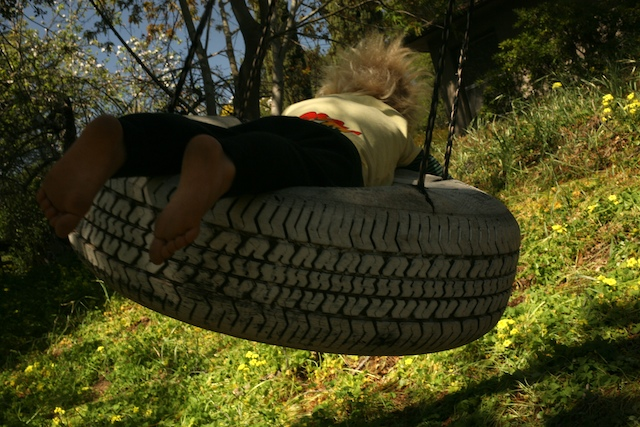Categories

# Pressure-volume work

The meaning of work in thermodynamics, and how to calculate work done by the compression or expansion of a gas.

## Key Points:

• Work is the energy required to move something against a force.
• The energy of a system can change due to work and other forms of energy transfer such as heat.
• Gases do expansion or compression work following the equation:work = −PΔV

## Introduction: Work and thermodynamics

When people talk about work in day-to-day conversation, they generally mean putting effort into something. You might “work on a school project” or “work to perfect your baseball pitch.” In thermodynamics, however, work has a very specific meaning: it is the energy it takes to move an object against a force. Work w, is one of the fundamental ways energy enters or leaves a system, and it has units of Joules (J).

When a system does work on the surroundings, the system’s internal energy decreases. When a system has work done on it, the internal energy of the system increases. Like heat, the energy change from work always occurs as part of a process: a system can do work, but doesn’t contain work.If the system is a child on a tire swing, we can do work on the system by giving it a push. We will be doing work against gravity, and this will increase the internal energy of the child on the swing,

To calculate work done by a constant force, we can use the following general equation:

work = force × displacementstart

For the purposes of chemistry class (as opposed to physics class), the most important takeaway from this equation is that work is proportional to the displacement as well as the magnitude of the force used. Different versions of the work equation can be used depending on the type of force involved. Some examples of doing work include:

• A person lifting books from the ground to a shelf does work against gravity.
• A battery pushing electrical current through a circuit does work against resistance.
• A child pushing a box along the floor does work against friction.

In thermodynamics, we are mainly interested in work done by expanding or compressing gases.